NEET  >  Resonance

# Resonance Notes | Study Physics Class 12 - NEET

## Document Description: Resonance for NEET 2022 is part of Physics Class 12 preparation. The notes and questions for Resonance have been prepared according to the NEET exam syllabus. Information about Resonance covers topics like and Resonance Example, for NEET 2022 Exam. Find important definitions, questions, notes, meanings, examples, exercises and tests below for Resonance.

Introduction of Resonance in English is available as part of our Physics Class 12 for NEET & Resonance in Hindi for Physics Class 12 course. Download more important topics related with notes, lectures and mock test series for NEET Exam by signing up for free. NEET: Resonance Notes | Study Physics Class 12 - NEET
 1 Crore+ students have signed up on EduRev. Have you?

8. Resonant Frequency

A series LCR circuit is said to be in the resonance condition when the current through it has its maximum value.

The current amplitude I0 for a series LCR circuit is given by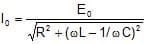Clearly I0 becomes zero both for ω → 0 and ω → ∞. The value of I0 is maximum when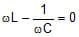or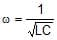⇒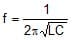Then impedance will be minimum

Zmin = R

The circuit is purely resistive. The current and voltage are in the same phase and the current in the circuit is maximum. This condition of the LCR circuit is called resonance condition.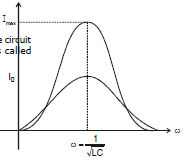The variance of I0 v/s ω shown in following figure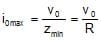So cos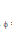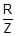=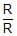= 1

V = V0 sin (ωt)

Impedance phase of resonance circuit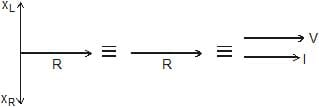Impedance of the circuit is minimum and heat generated in the circuit is maximum.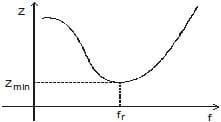Ex.15 In following LCR circuit find Z, i(t), VOC, VOL at resonace frequency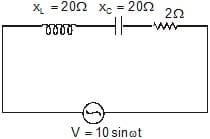Sol. Z = Zmin = R = 2?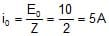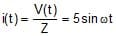VO L = i0X= 100 volt

VO L = i0 XL = 100 volt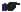: Above circuit is used as voltage amplifier (magnification) as peak value of voltage by source is only 10 while we can have maximum voltage up to 100 (VO C& VO L)

Ex.16 A series LCR with R = 20 ?, L = 1.5 H and C = 35 μF is connected to a variable frequency 200 V a.c. supply. When the frequency of the supply equals the natural frequency of the circuit. What is the average power transferred to the circuit in one complete cycle?

Sol. When the frequency of the supply equals the natural frequency of the circuit, resonance occurs.

Therefore, Z = R = 20 ohm

irms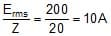Average power transferred/cycle

P = Ermsirms cos0° = 200 × 10 × 1 = 2000 watt

8.1 Sharpness of Resonance (Q - factor) :

The Q- factor of a series resonant circuit is defined as the ratio of the resonant frequency to the difference in two frequencies taken on the both sides of the resonant frequency such that at each frequency, the current amplitude becomes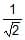times the value of resonant frequency.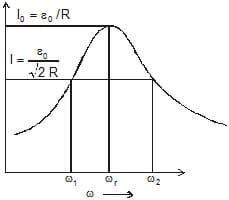Mathematically Q-factor.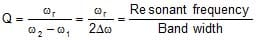or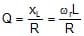9. Choke Coil :

A choke coil is simply an inductor with large inductance which is used to reduce current in a.c. circuit without much loss of energy.

Principle. A choke coil is based upon the principle that when a.c. flows through an inductor, the current lags behind the e.m.f. by a phase angle π/2.

Construction. A choke coil is basically an inductance. It consists of a large number of turns of insulated copper wire wound over a soft iron core. In order to minimize loss of electrical energy due to production of eddy currents, a laminated iron core is used.

In practice, a low frequency choke coil is made of insulated copper wire wound on a soft iron core, while a high frequency choke coil has air as core materials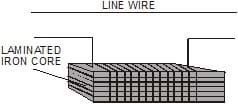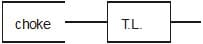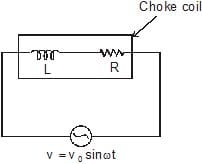Working : As shown in fig a choke is put in series across an electrical appliances of resistance R and is connected to an a.c. source.

Average power dissipiated per cycle in the circuit is

Pav = Veff Ieff cosf = Veff Ieff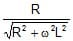.

Inductance L of the choke coil is very large so that R << wL. Then

Power factor cos φ ≌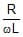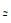0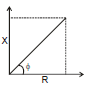tan φ =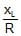Uses. In a.c. circuit, a choke coil is used to control the current in place of a resistance. If a resistance is used to control the current, the electrical energy will be wasted in the form of heat. A choke coil decreases the current without wasting electrical energy in the form of heat.

10. OSCILLATIONS IN L-C CIRCUIT

If a charged capacitor C is short-circuited through an inductor L, the charge and current in the circuit start oscillating simple harmonically. If the resistance of the circuit is zero, no energy is dissipated as heat. Assume an ideal situation in which energy is not radiated away from the circuit. With these idealizations-zero resistance and no radiation, the oscillations in the circuit persist indefinitely and the energy is transferred from capacitor's electric field to the inductor's magnetic field back and forth. The total energy associated with the circuit is constant. This is analogous to the transfer of energy in an oscillating mechanical system from potential energy to kinetic energy and back, with constant total energy. Such an analogous mechanical system is an example of spring mass system.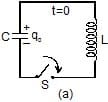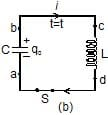Let us now derive an equation for the oscillations of charge and current in an L-C circuit. Refer figure (a) : The capacitor is charged to a potential difference V such that charge on capacitor q0 = CV

Here q0 is the maximum charge on the capacitor. At time t = 0, it is connected to an inductor through a switch S. At time t = 0, the switch S is closed.

Refer figure (b) : When the switch is closed, the capacitor starts discharging. Let at time t charge on the capacitor is q (<q0) and since, it is further decreasing, there is a current i in the circuit in the direction shown in figure.

The potential difference across capacitor = potential difference across inductor, or

Vb - Va = Vc - Vd

Therefore,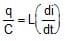...(1)

Now, as the charge is decreasing, i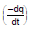or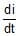= -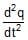Substituting in equation (1), we get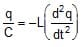or= -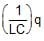...(2)

This is the standard equation of simple harmonic motion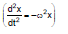Here w =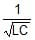...(3)

The general solution of equation (2), is

q = q0 cos (ωt ± φ) ...(4)

In our case φ = 0 as q = q0 at t = 0.

Thus, we can say that the charge in the circuit oscillates with angular frequency ω given by equation (3). Thus,

ln L - C oscillations, q, i andall oscillate simple harmonically with same angular frequency ω, but the phase difference between q and i or between i and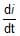is . Their amplitudes are q0 q0ω are ω2 q0 respectively. So

q = q0 cosωt, then ...(5)

i = -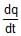= q0ω sin ωt ...(6)

and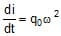cosωt ...(7)

Potential energy in the capacitor

UC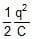=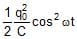...(8)

Potential energy in the inductor

UL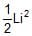=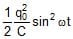...(9)

Thus potential energy stored in the capacitor and that in the inductor also oscillates between maximum value and zero with double the frequency. All these quantities are shown in the figures that follows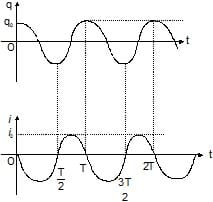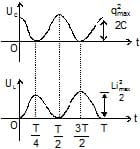Ex.17 A capacitor of capacitance 25 μF is charged to 300 v. It is then connected across a 10 μH inductor. The resistance of the circuit is negligible.

(a) Find the frequency of oscillation of the circuit.

(b) Find the potential difference across capacitor and magnitude of circuit current 1.2 ms after the inductor and capacitor are connected.

(c) Find the magnetic energy and electric energy at t = 0 and t = 1.2 ms.

Sol. (a) The frequency of oscillation of the circuit is ,

f =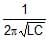Substituting the given values we have,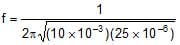=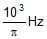(b) Charge across the capacitor at time t will be ,

q = q0 cos ωt

and i = - q0 ωsin ωt

Here q0 = CV0 = (25 × 10-6) (300) = 7.5 × 10-3 C

Now, charge is the capacitor after t = 1.2 × 10-3 s is,

q = (7.5 × 10-3) cos (2p × 318.3) (1.2 × 10-3)C

= 5.53 × 10-3C

Therefore, P.D. across capacitor,

V =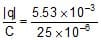= 221.2 volt

The magnitude of current in the circuit at

t = 1.2 × 10-3 s is,

|i| = q0 ω sinωt

= (7.5 × 10-3) (2p) (318.3) sin(2p × 318.3) (1.2 × 10-3) A = 10.13 A

(c) At t = 0 : Current in the circuit is zero. Hence,UL = 0

Charge on the capacitor is maximum

Hence, Uc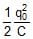or Uc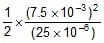= 1.125 J

Therefore, Total energy E = UL + U= 1.125 J

At t = 1.2 ms

UL=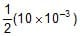(10.13)2 = 0.513 J

UC = E - UL = 1.125 - 0.513 = 0.612 J

Otherwise UC can be calculated as,

UC=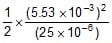= 0.612 J

The document Resonance Notes | Study Physics Class 12 - NEET is a part of the NEET Course Physics Class 12.
All you need of NEET at this link: NEET

## Physics Class 12

157 videos|425 docs|213 tests
 Use Code STAYHOME200 and get INR 200 additional OFF

## Physics Class 12

157 videos|425 docs|213 tests

### How to Prepare for NEET

Read our guide to prepare for NEET which is created by Toppers & the best Teachers

Track your progress, build streaks, highlight & save important lessons and more!

,

,

,

,

,

,

,

,

,

,

,

,

,

,

,

,

,

,

,

,

,

;